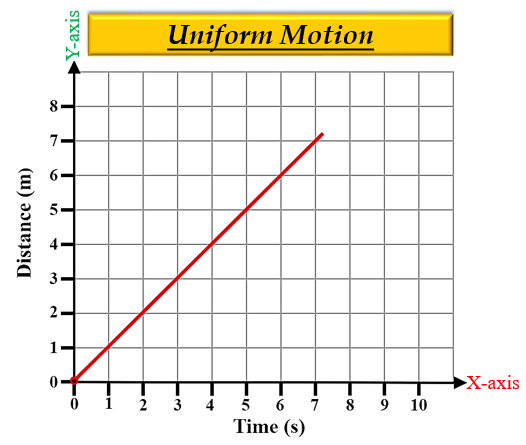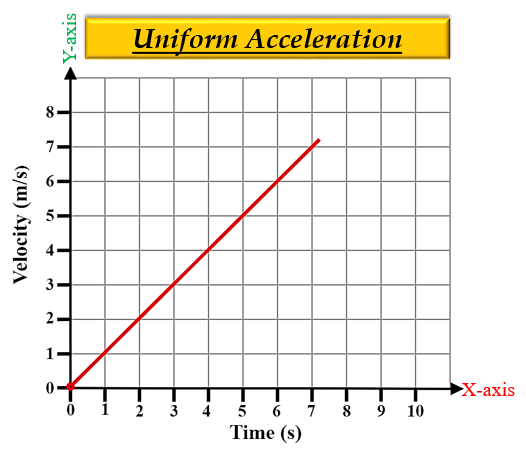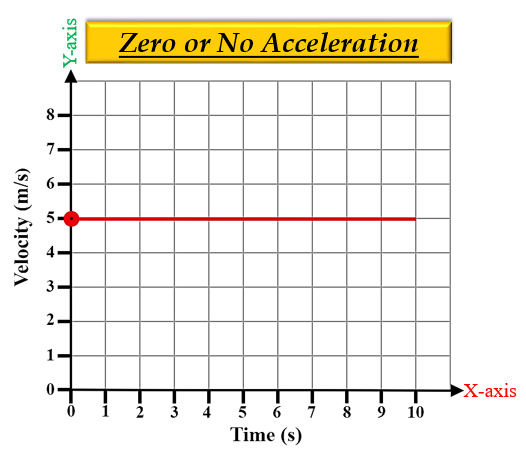# What does a straight line graph indicates?

In Distance-time graphs

If the graph is a straight line then the motion of the object is uniform, which implies that the speed of an object under uniform motion remains constant.

Uniform motion- A body is said to be in uniform motion if it travels equal distances in equal intervals of time, no matter how small these time intervals maybe. In other words, the distance travelled is directly proportional to time. Thus, a distance-time graph for uniform motion will be a straight line.In Velocity-time graphs with constant/uniform acceleration.

When the speed of the body changes at a uniform rate, its speed changes by equal amounts in equal intervals of time. So, the velocity-time graph of a body moving with uniform acceleration is a straight line.In Velocity-time graphs with constant/uniform velocity (zero acceleration).

When the speed of the body remains constant, then it means the speed of the body is not changing with respect to time and hence, there is zero or no acceleration.

So, the velocity-time graph of a body moving with constant speed is a straight line parallel to the x-axis (time axis).